# Common Core: High School - Geometry : Relationships Between Angles, Radii, Chords, and Diameters of Circles: CCSS.Math.Content.HSG-C.A.2

## Example Questions

← Previous 1

### Example Question #1 : Relationships Between Angles, Radii, Chords, And Diameters Of Circles: Ccss.Math.Content.Hsg C.A.2

If the arc measure is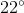what is the measure of the central angle?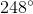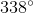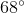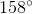Explanation:

The central angle is the same as the arc measure.

So the answer is### Example Question #2 : Relationships Between Angles, Radii, Chords, And Diameters Of Circles: Ccss.Math.Content.Hsg C.A.2

If the arc measure is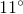what is the measure of the central angle?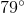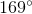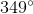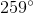Explanation:

The central angle is the same as the arc measure.

So the answer is### Example Question #1 : Relationships Between Angles, Radii, Chords, And Diameters Of Circles: Ccss.Math.Content.Hsg C.A.2

If the arc measure is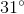what is the measure of the central angle?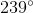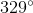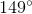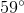Explanation:

The central angle is the same as the arc measure.

So the answer is### Example Question #4 : Relationships Between Angles, Radii, Chords, And Diameters Of Circles: Ccss.Math.Content.Hsg C.A.2

If the arc measure is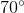what is the measure of the central angle?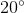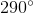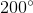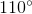Explanation:

The central angle is the same as the arc measure.

So the answer is### Example Question #5 : Relationships Between Angles, Radii, Chords, And Diameters Of Circles: Ccss.Math.Content.Hsg C.A.2

If the arc measure is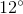what is the measure of the central angle?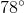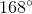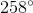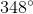Explanation:

The central angle is the same as the arc measure.

So the answer is### Example Question #1 : Relationships Between Angles, Radii, Chords, And Diameters Of Circles: Ccss.Math.Content.Hsg C.A.2

If the arc measure iswhat is the measure of the central angle?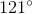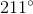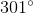Explanation:

The central angle is the same as the arc measure.

So the answer is### Example Question #1 : Relationships Between Angles, Radii, Chords, And Diameters Of Circles: Ccss.Math.Content.Hsg C.A.2

What is the measure of an inscribed angle with an arc measurement of?Explanation:

The inscribed angle is simply half the arc measurement.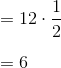### Example Question #1 : Relationships Between Angles, Radii, Chords, And Diameters Of Circles: Ccss.Math.Content.Hsg C.A.2

What is the measure of an inscribed angle with an arc measurement of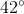.Explanation:

The inscribed angle is simply half the arc measurement.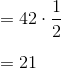### Example Question #1 : Relationships Between Angles, Radii, Chords, And Diameters Of Circles: Ccss.Math.Content.Hsg C.A.2

What is the measure of an inscribed angle with an arc measurement of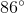?Explanation:

The inscribed angle is simply half the arc measurement.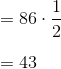### Example Question #6 : Relationships Between Angles, Radii, Chords, And Diameters Of Circles: Ccss.Math.Content.Hsg C.A.2

What is the measure of an inscribed angle with an arc measurement of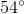?Explanation:

The inscribed angle is simply half the arc measurement.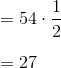← Previous 1

### All Common Core: High School - Geometry Resources